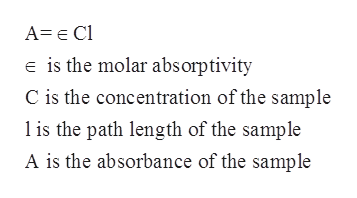# a.) The molar absorption coefficient of a substance dissolved in benzene is measured to be 802 L mol-1 cm-1 at 492 nm. Calculate what fraction of the incident light intensity of that wavelength passes through 2.97 mm of a solution of concentration 3.06 mmol/L.b.) The molar absorption coefficient of a solute at 524 nm is 103 dm3 mol-1 cm-1. When light of that wavelength passes through a 4.70 mm cell containing a solution of the solute, 59.4 per cent of the light was absorbed. What is the concentration of the solution?Ans = ______ mmol dm-3

Question
10 views

a.) The molar absorption coefficient of a substance dissolved in benzene is measured to be 802 L mol-1 cm-1 at 492 nm. Calculate what fraction of the incident light intensity of that wavelength passes through 2.97 mm of a solution of concentration 3.06 mmol/L.

b.) The molar absorption coefficient of a solute at 524 nm is 103 dm3 mol-1 cm-1. When light of that wavelength passes through a 4.70 mm cell containing a solution of the solute, 59.4 per cent of the light was absorbed. What is the concentration of the solution?
Ans = ______ mmol dm-3

check_circle

Step 1

The term absorbance refers to the measure of the quantity of light which has been absorbed by the sample.

Step 2

According to the Beer Lambert law, the absorbance of a given solution is directly proportional to the concentration and the pathlength of the sample. The mathematical formula is given as follows:help_outlineImage TranscriptioncloseA= e Cl e is the molar absorptivity C is the concentration of the sample is the path length of the sample A is the absorbance of the sample fullscreen
Step 3

The path length of the solution in the given problem is given to be 2.97 mm=0.297 cm.

The molar absorptivity of the sample of benzene is given to be 802 L mol-1cm-1.

The c...

### Want to see the full answer?

See Solution

#### Want to see this answer and more?

Solutions are written by subject experts who are available 24/7. Questions are typically answered within 1 hour.*

See Solution
*Response times may vary by subject and question.
Tagged in

### Physical Chemistry